# Metric Volume

These are the two most common measurements:

• Milliliters
• Liters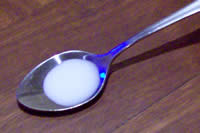A milliliter (that is "milli" and "liter" put together) is a very small amount of liquid.

Here is a milliliter of milk in a teaspoon.

It doesn't even fill the teaspoon!

 If you collect about 20 drops of water, you will have 1 milliliter: 20 drops of watermakes about 1 milliliter And a teaspoon can hold about five milliliters: 1 teaspoon of liquid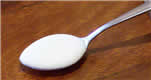is about 5 milliliters

###Milliliters are often written as ml (for short), so "100 ml" means "100 milliliters".

Here we have 150 ml of milk in a measuring cup.

It doesn't say "150" ... it says "50" ... but it is half-way between 100 and 200 so you can figure out it is 150 ml.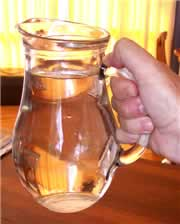## Liter

liter is just a bunch of milliliters put all together. In fact, 1000 milliliters makes up 1 liter:

1 liter = 1.000 milliliters

This jug has exactly 1 liter of water in it.

Liters are often written as L (for short), so "3 L" means "3 Liters" (some people use lowercase l, but that looks too much like 1).

Milk, soda and other drinks are often sold in liters.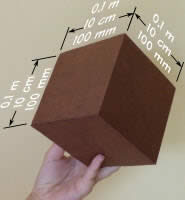A cube that is 0,1 meters (10 cm or 100 mm)  on each side contains 1 liter,
 and a square meter that is 1 millimeter thick also contains 1 liter.The next time you are at the store take a minute and look on the labels to see how many liters (or milliliters) are in each container!

## Other Volume Measurements

You may come across these other volume measurements:

### Cubic Meter (m3)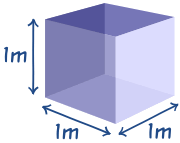Volume is length by length by length, so the basic unit of volume is a cube that is 1 meter on each side.

The Unit is written m3 (cubic meters).

So, a cube that is 1 meter on each side is a cubic meter (m3) ...

... and that is also equal to 1.000 liters.

1 m3 = 1.000 Liters

### Megaliters (ML)

Megaliter is a million liters (1.000.000 L). Useful for measuring large quantities of water, such as in lakes and dams.

### Cubic Centimeter (cc)

A Cubic Centimeter (abbreviated cc or cm3) is a cube that is 1 centimeter on each side.

It equals 1 mL (one-thousandth of a liter), and one-millionth of a cubic meter. Or to put it another way:

1 m3 = 1.000 L = 1.000.000 cm3
(one cubic meter = a thousand liters = a million cubic centimeters)

### Cubic Millimeter

A Cubic Millimeter is a cube that is 1 millimeter on each side.

It is one-millionth of a liter, and one-billionth of a cubic meter.

It is very small, and we need 1.000 Cubic Millimeters to make just one milliliter.

## More Examples

A liter (L) is:

• a bit more than a quart (US measure)
• about the quantity in a sports drink bottle
• close to the volume of a kilogram of water